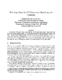## Fast Algorithms for 3-D Dominance Reporting and Counting##### Files
CS-TR-4437.ps(186.76 KB)
We present in this paper fast algorithms for the 3-D dominance reporting and counting problems, and generalize the results to the d-dimensional case. Our 3-D dominance reporting algorithm achieves $O(\log n/\log\log n +f)$ query time using $O(n\log^{\epsilon}n)$ space, where $f$ is the number of points satisfying the query and $\epsilon>0$ is an arbitrary small constant. For the 3-D dominance counting problem (which is equivalent to the 3-D range counting problem), our algorithm runs in $O((\log n/\log\log n)^2)$ time using $O(nlog^{1+\epsilon}n/\log\log n)$ space. Also UMIACS-TR-2003-06